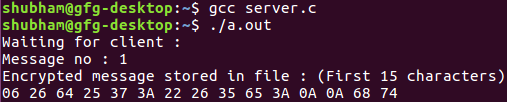Open in App
Not now

# Message encryption and decryption using UDP server

• Difficulty Level : Expert
• Last Updated : 26 Jul, 2021

The process of message encryption and decryption during client-server communication using UDP server is as follows:

• The client requests the server with a file name.
• The corresponding file is opened by the server and sends the file using datagram socket.
• The sender sends the encrypted text (Xoring) with a fixed length key.
• The receiver decrypts the file using the same key (private key).

Prerequisite : Socket Programming

Examples:

```Input :  file.txt
Output : ClientSide : Received from server:
00 2C 28 28 2A 6E 3B 24 37 64 65 79 0A 00 00
Decrypted message: Hello People
ServerSide : Encrypted message stored in file:
00 2C 28 28 2A 6E 3B 24 37 64 65 79 0A 00  ```

Client Side Program:

## C

 `// Client side code` `#include ` `#include ` `#include ` `#include `   `// Driver code` `int` `main()` `{` `    ``int` `clientSocket, portNum, nBytes;` `    ``char` `file_buffer, path, buffer;`   `    ``// This key array stores the hidden key` `    ``char` `const` `key = ``"HIDDENKEY"``;` `    ``struct` `sockaddr_in serverAddr;` `    ``socklen_t addr_size;` `    ``int` `i;` `    ``clientSocket = socket(PF_INET, SOCK_DGRAM, 0);`   `    ``serverAddr.sin_family = AF_INET;` `    ``serverAddr.sin_port = htons(5004);` `    ``serverAddr.sin_addr.s_addr = inet_addr(``"127.0.0.1"``);` `    ``memset``(serverAddr.sin_zero, ``'\0'``, ``sizeof` `serverAddr.sin_zero);`   `    ``addr_size = ``sizeof` `serverAddr;`   `    ``while` `(1) {` `        ``printf``(``"Specify file name: \n"``);` `        ``gets``(path);`   `        ``// printf("%s\n", path);` `        ``FILE``* fp;` `        ``fp = ``fopen``(path, ``"r"``);` `        ``if` `(fp == NULL) {` `            ``printf``(``"file does not exist\n"``);` `        ``}`   `        ``fseek``(fp, 0, SEEK_END);` `        ``size_t` `file_size = ``ftell``(fp);` `        ``fseek``(fp, 0, SEEK_SET);`   `        ``if` `(``fread``(file_buffer, file_size, 1, fp) <= 0) {` `            ``printf``(``"unable to copy file into buffer\n"``);` `            ``exit``(1);` `        ``}`   `        ``if` `(sendto(clientSocket, file_buffer, 3000, 0, (``struct` `sockaddr*)&serverAddr, ` `                                                                    ``addr_size) < 0) {` `            ``printf``(``"error in sending the file\n"``);` `            ``exit``(1);` `        ``}`   `        ``bzero(file_buffer, ``sizeof``(file_buffer));`   `        ``nBytes = recvfrom(clientSocket, buffer, 1024, 0, NULL, NULL);`   `        ``printf``(``"Received from server: \n"``);`   `        ``// printing some of the character to have a feel of encryption` `        ``for` `(i = 0; i < 15; ++i)` `            ``printf``(``"%02X "``, buffer[i]);` `        ``printf``(``"\n"``);`   `        ``char` `x;` `        ``for` `(i = 0; i < nBytes - 1; ++i)` `            ``x[i] = (``char``)(buffer[i] ^ key[i]);`   `        ``// printing some of the character to have a feel of decryption` `        ``printf``(``"Decrypted message: (First 15 characters)\n"``);` `        ``for` `(i = 0; i < 11; ++i)` `            ``printf``(``"%c "``, x[i]);`   `        ``printf``(``"\n"``);` `    ``}`   `    ``return` `0;` `}`

Server Side Program:

## C

 `// C server code` `#include ` `#include ` `#include ` `#include ` `#include ` `#include `   `// Driver code` `int` `main()` `{` `    ``int` `udpSocket, nBytes;` `    ``char` `buffer, xor;` `    ``char` `const` `key = ``"HIDDENKEY"``;` `    ``struct` `sockaddr_in serverAddr, clientAddr;` `    ``struct` `sockaddr_storage serverStorage;` `    ``socklen_t addr_size, client_addr_size;` `    ``int` `i;`   `    ``udpSocket = socket(PF_INET, SOCK_DGRAM, 0);`   `    ``serverAddr.sin_family = AF_INET;` `    ``serverAddr.sin_port = htons(5004);` `    ``serverAddr.sin_addr.s_addr = inet_addr(``"127.0.0.1"``);` `    ``memset``(serverAddr.sin_zero, ``'\0'``, ``sizeof` `serverAddr.sin_zero);`   `    ``bind(udpSocket, (``struct` `sockaddr*)&serverAddr, ``sizeof``(serverAddr));`   `    ``addr_size = ``sizeof` `serverStorage;` `    ``puts``(``"Waiting for client :"``);` `    ``int` `count = 0;`   `    ``while` `(1) {` `        ``nBytes = recvfrom(udpSocket, buffer, 3000, 0, (``struct` `sockaddr*)&serverStorage, ` `                                                                            ``&addr_size);` `        ``printf``(``"Message no : %d\n"``, ++count);`   `        ``for` `(i = 0; i < nBytes - 1; i++) {` `            ``if` `(buffer[i] != ``'\n'``)` `                ``xor[i] = (``char``)(buffer[i] ^ key[i]);` `            ``else` `                ``xor[i] = buffer[i];` `        ``}`   `        ``printf``(``"Encrypted message stored in file : (First 15 characters)\n"``);`   `        ``// printing some of the character to have a feel of encryption` `        ``for` `(i = 0; i < 15; ++i)` `            ``printf``(``"%02X "``, xor[i]);` `        ``printf``(``"\n"``);`   `        ``FILE``* fp;` `        ``fp = ``fopen``(``"temp.txt"``, ``"w+"``);`   `        ``for` `(i = 0; i < nBytes - 1; i++) {` `            ``if` `(xor[i] != ``'\n'``)` `                ``fprintf``(fp, ``"%X"``, xor[i]);` `            ``else` `                ``fprintf``(fp, ``"%c"``, xor[i]);` `        ``}`   `        ``fclose``(fp);` `        ``sendto(udpSocket, xor, nBytes, 0, (``struct` `sockaddr*)&serverStorage, ` `                                                                 ``addr_size);` `    ``}`   `    ``return` `0;` `}`

OutputMy Personal Notes arrow_drop_up
Related Articles